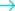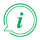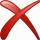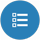# Convert speed from Metre per hour to Metre per second## Convert from Metre per hour

### About Metre per hour to Metre per second converter

##### Info

To convert from Metre per hour to Metre per second fill in the conversion tool field with the amount you want to convert. The result of Metre per hour to Metre per second conversion will be appeared in the "Result" field in red characters, without need of pressing any button. Below the conversion tool, a list will appear with all the available conversions from Metre per hour.

#### Examples of Common Queries about converting Metre per hour to Metre per second

##### Metre per hour to Metre per second converter helps you to find a solution about:
• How do I turn Metre per hour into Metre per second?
• How to convert Metre per hour to Metre per second.
• How to make Metre per hour Metre per second.
• How do I convert Metre per hour speed to Metre per second speed ?
• Is Metre per hour to Metre per second converter free?
• Where can i find Metre per hour to Metre per second converter online.
• Is there a way to convert Metre per hour to Metre per second?